Home MATHEMATICS TOPIC 1: NUMBERS ~ MATHEMATICS FORM 1

# TOPIC 1: NUMBERS ~ MATHEMATICS FORM 1

3829
9
SHARE#### NUMBERS

We know that when we count we start 1,2 …. . But there are other numbers

like 0, negative numbers and decimals. All these types of numbers are
categorized in different groups like counting numbers, integers,real
numbers, whole numbers and rational and irrational numbers according to
their properties. all this have been covered in this chapter
Numbers
are represented by symbols called numerals. For example, numeral for
the number ten is 10. Numeral for the number hundred is 110 and so on.
The
symbols which represent numbers are called digits. For example the
number 521 has three (3) digits which are 5, 2 and 1. There are only
tendigits which are used to represent any number. These digits are 0, 1,
2, 3, 4, 5, 6, 7, 8, and 9.
The Place Value in each Digit in Base Ten Numeration
Identify the place value in each digit in base ten numeration
When
we write a number, for example 521, each digit has a different value
called place value. The 1 on the right means 1 ones which can be written
as 1 × 1, the next number which is 2 means 2 tens which can be written
as 2 × 10 and the last number which is 5 means 5 hundreds which can be
written as 5 × 100. Therefore the number 521 was found by adding the
numbers 5 × 100 + 2 × 10 + 1× 1 = 521.
Note that when writing numbers in words, if there is zero between numbers we use word ‘and’
Example 1
Write the following numbers in words:
1. 7 008
2. 99 827 213
3. 59 000
Solution
1. 7 008 = Seven thousand and eight.
2. 99 827 213 = Ninety nine millions eight hundred twenty seven thousand two hundred thirteen.
3. 59 000 = Fifty nine thousand.
Example 2
Write the numbers bellow in expanded form.
1. 732.
2. 1 205.
Solution
1. 732 = 7 x 100 + 3 x 10 + 2 x 1
2. 1 205 = 1 x 1000 + 2 x 100 + 0 x 10 + 5 x 1
Example 3
Write in numerals for each of the following:
1. 9 x 100 + 8 x 10 + 0 x 1
2. Nine hundred fifty five thousand and five.
Solution
1. 9 x 100 + 8 x 10 + 0 x 1 = 980
2. Nine hundred fifty five thousand and five = 955 005.
Example 4
For each of the following numbers write the place value of the digit in brackets.
1. 89 705 361 (8)
2. 57 341 (7)
Solution
1. 8 is in the place value of ten millions.
2. 7 is in the place value of thousands.
Numbers in Base Ten Numeration
Read numbers in base ten numeration
Base
Ten Numeration is a system of writing numbers using ten symbols i.e. 0,
1, 2, 3, 4, 5, 6, 7, 8 and 9. Base Ten Numeration is also called
decimal system of Numeration.
Numbers in Base Ten Numeration up to One Billion
Write numbers in base ten numeration up to one billion
Consider the table below showing place values of numbers up to one Billion.
 Billions Hundred millions Ten millions Millions Hundred Thousands Ten Thousands Thousands Hundreds Tens Ones 1 1 0 1 0 0 1 0 0 0 1 0 0 0 0 1 0 0 0 0 0 1 0 0 0 0 0 0 1 0 0 0 0 0 0 0 1 0 0 0 0 0 0 0 0 1 0 0 0 0 0 0 0 0 0
If
you are given numerals for a number having more than three digits, you
have to write it by grouping the digits into groups of three digits from
right. For example 7892939 is written as 7 892 939.
When
we are writing numbers in words we consider their place values. For
example; if we are told to write 725 in words, we first need to know the
place value of each digit. Starting from right side 5 is in the place
value of ones, 2 is in the place value of tens and seven is in the place
value of hundreds. Therefore our numeral will be read as seven hundred
twenty five.
Numbers in Daily Life
Apply numbers in daily life
Numbers
play an important role in our lives. Almost all the things we do
involve numbers and Mathematics. Whether we like it or not, our life
revolves in numbers since the day we were born. There are numerous
numbers directly or indirectly connected to our lives.
The following are some uses of numbers in our daily life:
1. Calling a member of a family or a friend using mobile phone.
2. Calculating your daily budget for your food, transportation, and other expenses.
3. Cooking, or anything that involves the idea of proportion and percentage.
4. Weighing fruits, vegetables, meat, chicken, and others in market.
5. Using elevators to go places or floors in the building.
6. Looking at the price of discounted items in a shopping mall.
7. Looking for the number of people who liked your post on Facebook.
8. Switching the channels of your favorite TV shows.
9. Telling time you spent on work or school.
Integers
Identify integers
Consider a number line below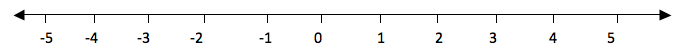The
numbers from 0 to the right are called positive numbers and the numbers
from 0 to the left with minus (-) sign are called negative numbers.
Therefore all numbers with positive (+) or negative (-) sign are called
integers and they are denoted by Ζ. Numbers with positive sign are
written without showing the positive sign. For example +1, +2, +3, …
they are written simply as 1, 2, 3, … . But negative numbers must carry
negative sign (-). Therefore integers are all positive and negative
numbers including zero (0). Zero is neither positive nor negative
number. It is neutral.
The
numbers from zero to the right increases their values as the increase.
While the numbers from zero to the left decrease their values as they
increase. Consider a number line below.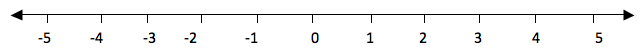If
you take the numbers 2 and 3, 3 is to the right of 2, so 3 is greater
than 2. We use the symbol ‘>’ to show that the number is greater than
i. e. 3 >2(three is greater than two). And since 2 is to the left of
3, we say that 2 is smaller than 3 i.e. 2<3. The symbol ‘<’ is
use to show that the number is less than.
Consider
numbers to the left of 0. For example if you take -5 and -3. -5 is to
the left of -3, therefore -5 is smaller than -3. -3 is to the right of
-5, therefore -3 is greater than -5.
Generally, the number which is to the right of the other number is greater than the number which is to the left of it.
If
two numbers are not equal to each to each other, we use the symbol ‘≠’
to show that the two numbers are not equal. The not equal to ‘≠’ is the
opposite of is equal to ‘=’.
Example 21
Represent the following integers Ζ on a number line
1. 0 is greater than Ζ and Ζ is greater than -4
2. -2 is less than Ζ and Ζ is less than or equal to 1.
Solution
a.
0 is greater than Ζ means the integers to the left of zero and Ζ is
greater than -4 means integers to the left of -4. These numbers are -1,
-2 and -3. Consider number line below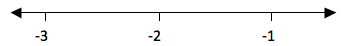b.
-2 is less than Ζ means integers to the right of -2 and Ζ is less than
or equal to 1 means integers to the left of 1 including 1. These
integers are -1, 0 and 1. Consider the number line below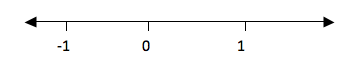Example 22
Put the signs ‘is greater than’ (>), ‘is less than’ (<), ‘is equal to’ (=) to make a true statement.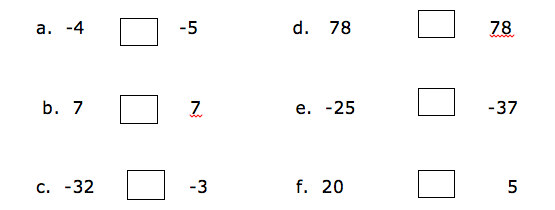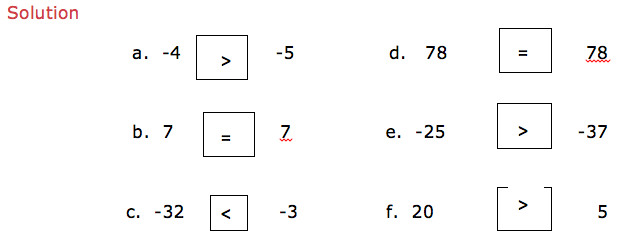Example 23
2 + 3
Show a picture of 2 and 3 on a number line.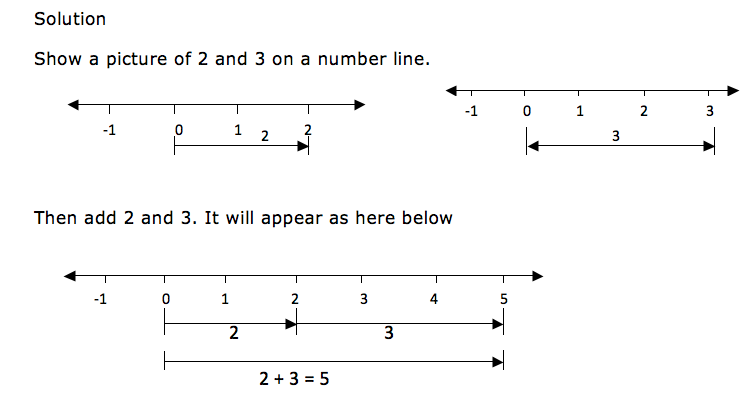When
drawing integers on a number line, the arrows for the positive numbers
goes to the right while the arrows for the negative numbers goes to the
left. Consider an illustration bellow.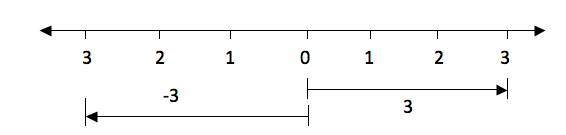The
distance from 0 to 3 is the same as the distance from 0 to -3, only the
directions of their arrows differ. The arrow for positive 3 goes to the
right while the arrow for the negative 3 goes to the left.
Example 24
-3 + 6
Solution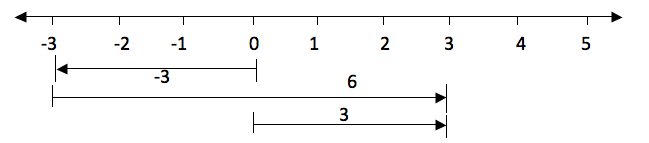Subtraction of Integers
Subtract integers
Since
subtraction is the opposite of addition, if for example you are given
5-4 is the same as 5 + (-4). So if we have to subtract 4 from 5 we can
use a number line in the same way as we did in addition. Therefore 5-4
on a number line will be: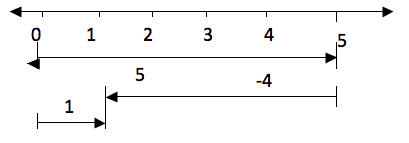Take five steps from 0 to the right and then four steps to the left from 5. The result is 1.
Multiplication of Integers
Multiply integers
Example 25
2×6 is the same as add 2 six times i.e. 2×6 = 2 + 2 + 2 + 2 + 2 +2 = 12. On a number line will be: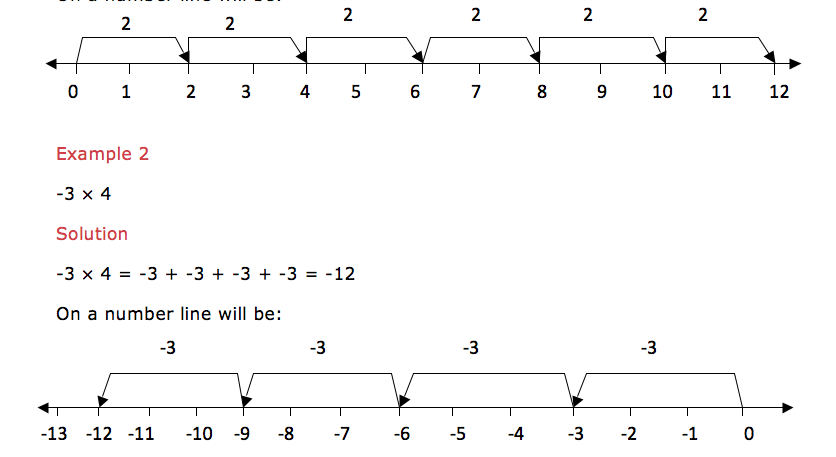Multiplication
of a negative integer by a negative integer cannot be shown on a number
line but the product of these two negative integers is a positive
integer.
From
the above examples we note that multiplication of two positive integers
is a positive integer. And multiplication of a positive integer by a
negative integer is a negative integer. In summary:
• (+)×,(+) = (+)
• (-)×,(-) = (+)
• (+)×,(-) = (-)
• (-)×,(+) = (-)
Division of Integers
Divide integers
Example 26
6÷3 is the same as saying that, which number when you multiply it by 3 you will get 6, that number is 2, so, 6÷3 = 2.
Therefore
division is the opposite of multiplication. From our example 2×3 = 6
and 6÷3 = 2. Thus multiplication and division are opposite to each
other.
Dividing
two integers which are both positive the quotient (answer) is a
positive integer. If they are both negative also the quotient is
positive. If one of the integer is positive and the other is negative
then the quotient is negative. In summary:
• (+)÷(+) = (+)
• (-)÷(-) = (+)
• (+)÷(-) = (-)
• (-)÷(+) = (-)
Mixed Operations on Integers
Perform mixed operations on integers
You
may be given more than one operation on the same problem. Do
multiplication and division first and then the rest of the signs. If
there are brackets, we first open the brackets and then we do division
followed by multiplication, addition and lastly subtraction. In short we
call it BODMAS. The same as the one we did on operations on whole
numbers.
Example 27
9÷3 + 3×2 -1 =
Solution
9÷3 + 3×2 -1
=3 + 6 -1 (first divide and multiply)
Example 28
(12÷4 -2) + 4 – 7=
Solution
(12÷4 -2) + 4 – 7
=1 + 4 – 7 (do operations inside the brackets and divide first)
=2

1. Wonderful, what a web site it is! This blog gives helpful facts to us, keep it up.

2. Magnificent goods from you, man. I have understand your stuff previous
to and you are just too excellent. I actually like what you’ve acquired here,
certainly like what you’re saying and the way in which you say
it. You make it enjoyable and you still take care of to keep it
smart. I can’t wait to read far more from you. This is really a
tremendous site.

3. Hey there! I’m at work browsing your blog from my new apple
iphone! Just wanted to say I love reading through your blog and look forward to
all your posts! Keep up the great work!

4. I visited several websites except the audio quality for audio songs existing at
this website is in fact marvelous. 0mniartist asmr

5. It’s an amazing post designed for all the internet
users; they will take benefit from it I am sure. asmr 0mniartist

6. Hello there! I just want to offer you a big thumbs up for your great info
you’ve got here on this post. I will be coming back to your web site for
more soon. 0mniartist asmr

7. Woah! I’m really digging the template/theme of this blog.
It’s simple, yet effective. A lot of times it’s tough to get that “perfect balance” between usability and appearance.
I must say that you’ve done a very good job with
9.Dani